• java保留两位小数5种方法 四舍五入，例： import java.math.BigDecimal; import java.text.DecimalFormat; import java.text.NumberFormat; public class format { double f = 111231.5585; public void m1() {...
java保留两位小数5种方法 四舍五入，例：
import java.math.BigDecimal;
import java.text.DecimalFormat;
import java.text.NumberFormat;

public class format {
double f = 111231.5585;

/**
* BigDecimal转double 四舍五入保留两位小数
*/
public void m1() {
BigDecimal bg = new BigDecimal(f);
double f1 = bg.setScale(2, BigDecimal.ROUND_HALF_UP).doubleValue();
System.out.println(f1);
}
/**
* BigDecimal除法 四舍五入保留四位小数
*/
public void m2() {
BigDecimal number = new BigDecimal(smsIncome).divide(new BigDecimal(smsIncomeChina), 4, RoundingMode.HALF_UP)
}
/**
* DecimalFormat转换最简便
*/
public void m3() {
DecimalFormat df = new DecimalFormat("#.00");
System.out.println(df.format(f));
}
/**
* String.format打印最简便
*/
public void m4() {
System.out.println(String.format("%.2f", f));
}
public void m5() {
NumberFormat nf = NumberFormat.getNumberInstance();
nf.setMaximumFractionDigits(2);
System.out.println(nf.format(f));
}

public static void main(String[] args) {
format f = new format();
f.m1();
f.m2();
f.m3();
f.m4();
f.m5();
}
}

展开全文• bigdecimal保留两位小数 public class test1_format { public static void main(String[] args) { BigDecimal decimal = new BigDecimal(“1.12345”); System.out.println(decimal); BigDecimal setScale = decimal...
bigdecimal保留两位小数
public class test1_format { public static void main(String[] args) { BigDecimal decimal = new BigDecimal(“1.12345”); System.out.println(decimal); BigDecimal setScale = decimal.setScale(4,BigDecimal.ROUND_HALF_DOWN); System.out.println(setScale);
BigDecimal setScale1 = decimal.setScale(4,BigDecimal.ROUND_HALF_UP); System.out.println(setScale1); } }
参数定义
ROUND_CEILING Rounding mode to round towards positive infinity. 向正无穷方向舍入
ROUND_DOWN Rounding mode to round towards zero. 向零方向舍入
ROUND_FLOOR Rounding mode to round towards negative infinity. 向负无穷方向舍入
ROUND_HALF_DOWN Rounding mode to round towards “nearest neighbor” unless both neighbors are equidistant, in which case round down. 向（距离）最近的一边舍入，除非两边（的距离）是相等,如果是这样，向下舍入, 例如1.55 保留一位小数结果为1.5
ROUND_HALF_EVEN Rounding mode to round towards the “nearest neighbor” unless both neighbors are equidistant, in which case, round towards the even neighbor. 向（距离）最近的一边舍入，除非两边（的距离）是相等,如果是这样，如果保留位数是奇数，使用ROUND_HALF_UP ，如果是偶数，使用ROUND_HALF_DOWN
ROUND_HALF_UP Rounding mode to round towards “nearest neighbor” unless both neighbors are equidistant, in which case round up. 向（距离）最近的一边舍入，除非两边（的距离）是相等,如果是这样，向上舍入, 1.55保留一位小数结果为1.6
ROUND_UNNECESSARY Rounding mode to assert that the requested operation has an exact result, hence no rounding is necessary. 计算结果是精确的，不需要舍入模式
ROUND_UP Rounding mode to round away from zero. 向远离0的方向舍入
展开全文java 人工智能 大数据 聚类
• BigDecimal保留两位小数及格式化成百分比在项目中经常会用到小数的一些计算，而float和double类型的主要设计目标是为了科学计算和工程计算。他们执行二进制浮点运算，这是为了在广域数值范围上提供较为精确的快速...
BigDecimal保留两位小数及格式化成百分比
在项目中经常会用到小数的一些计算，而float和double类型的主要设计目标是为了科学计算和工程计算。他们执行二进制浮点运算，这是为了在广域数值范围上提供较为精确的快速近似计算而精心设计的。然而，它们没有提供完全精确的结果，所以不应该被用于要求精确结果的场合。但是，商业计算往往要求结果精确。所以有时候必须要采用BigDecimal。
public class Demo {   public static void main(String[] args) {  BigDecimal a =null;  Integer faultRate = 6;  a = BigDecimal.valueOf(faultRate.doubleValue()/3);  BigDecimal  b =a.setScale(2, RoundingMode.HALF_UP);//保留两位小数  System.out.println("结果是"+b);  //下面将结果转化成百分比  NumberFormat percent = NumberFormat.getPercentInstance();       percent.setMaximumFractionDigits(2);       System.out.println(percent.format(b.doubleValue()));  }}
运行结果是：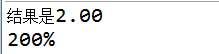BigDecimal.setScale()方法用于格式化小数点setScale(1)表示保留一位小数，默认用四舍五入方式 setScale(1,BigDecimal.ROUND_DOWN)直接删除多余的小数位，如2.35会变成2.3 setScale(1,BigDecimal.ROUND_UP)进位处理，2.35变成2.4 setScale(1,BigDecimal.ROUND_HALF_UP)四舍五入，2.35变成2.4
setScaler(1,BigDecimal.ROUND_HALF_DOWN)四舍五入，2.35变成2.3，如果是5则向下舍
setScaler(1,BigDecimal.ROUND_CEILING)接近正无穷大的舍入
setScaler(1,BigDecimal.ROUND_FLOOR)接近负无穷大的舍入，数字>0和ROUND_UP作用一样，数字<0和ROUND_DOWN作用一样
setScaler(1,BigDecimal.ROUND_HALF_EVEN)向最接近的数字舍入，如果与两个相邻数字的距离相等，则向相邻的偶数舍入。

注释：

1：scale指的是你小数点后的位数。比如123.456则score就是3.

score()就是BigDecimal类中的方法啊。比如:BigDecimal b = new BigDecimal("123.456");

b.scale(),返回的就是3.

2：roundingMode是小数的保留模式。它们都是BigDecimal中的常量字段,有很多种。比如：BigDecimal.ROUND_HALF_UP表示的就是4舍5入。3：pubilc BigDecimal divide(BigDecimal divisor, int scale, int roundingMode)的意思是说：我用一个BigDecimal对象除以divisor后的结果，并且要求这个结果保留有scale个小数位，roundingMode表示的就是保留模式是什么，是四舍五入啊还是其它的，你可以自己选！

BigDecimal mData = new BigDecimal("9.655").setScale(2, BigDecimal.ROUND_HALF_UP);        System.out.println("mData=" + mData);

----结果：----- mData=9.66


展开全文• 2、BigDecimal初始化赋值3、BigDecimal的加减乘除运算4、BigDecimal比较大小5、BigDecimal保留两位小数及舍入模式6、BigDecimal其他方法及常量 1、为什么要用BigDecimal ？ 工作中我们通过浮点数进行运算时，好像时...


文章目录
1、为什么要用BigDecimal ？2、BigDecimal初始化赋值3、BigDecimal的加减乘除运算4、BigDecimal比较大小5、BigDecimal保留两位小数及舍入模式6、BigDecimal其他方法及常量

1、为什么要用BigDecimal ？
工作中我们通过浮点数进行运算时，好像时不时的会出现一些小误差。例如：
public static void main(String[] args) {
System.out.println(1.9 - 1.2);
System.out.println(1.9 - 1.5);
System.out.println(100 - 99.8);
}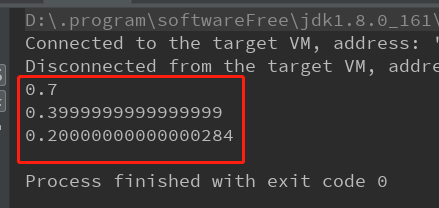大致搜了一下原因，网上说我们的计算机是二进制的，而浮点数是没有办法通过二进制精准的表示出来。 也就导致在运算的时候，float类型和double类型很容易丢失精度。 所以在开发中，如果我们需要精确计算的结果，可以使用java.math包中提供的BigDecimal类来进行操作。
2、BigDecimal初始化赋值
方法类型描述public BigDecimal(int val)构造函数int类型的值生成BigDecimal对象public BigDecimal(long val)构造函数long类型的值生成BigDecimal对象public BigDecimal(String val)静态方法String类型的值转换为BigDecimal类型public static BigDecimal valueOf(double val)静态方法double类型的值转换为BigDecimal类型public static BigDecimal valueOf(long val)静态方法long类型（包含int类型）的值转换为BigDecimal类型
代码示例：
BigDecimal b = new BigDecimal("33");
BigDecimal c = BigDecimal.valueOf(4.7);

注意：不建议使用public BigDecimal(double val)方式初始化值，编码时idea提示禁止使用构造方法BigDecimal(double)，描述如下：
使用了new BigDecimal(double)构造函数 less... (Ctrl+F1)
Inspection info:
禁止使用构造方法BigDecimal(double)的方式把double值转化为BigDecimal对象 说明：反编译出的字节码文件显示每次循环都会new出一个StringBuilder对象，然后进行append操作，最后通过toString方法返回String对象，造成内存资源浪费。

Negative example(不建议使用):
BigDecimal good1 = new BigDecimal(0.1);

Positive example(建议使用):
BigDecimal good1 = new BigDecimal("0.1");
BigDecimal good2 = BigDecimal.valueOf(0.1);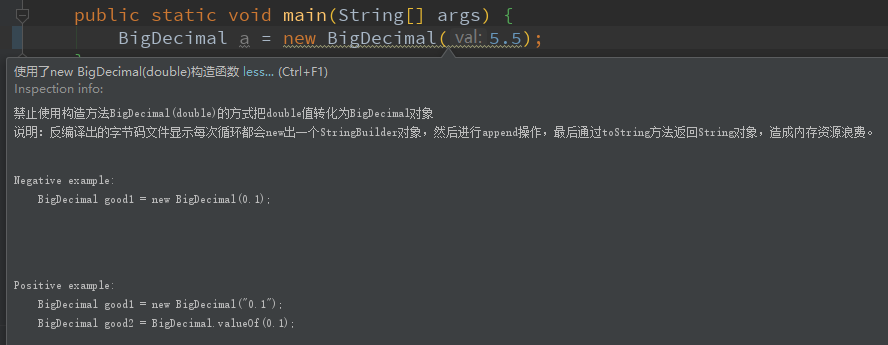3、BigDecimal的加减乘除运算
运算法则对应方法加法public BigDecimal add(BigDecimal value)减法public BigDecimal subtract(BigDecimal value)乘法public BigDecimal multiply(BigDecimal value)除法public BigDecimal divide(BigDecimal value)
代码示例：
public static void main(String[] args) {
System.out.println("计算减法: " + BigDecimal.valueOf(1.9).subtract(BigDecimal.valueOf(1.5)));
System.out.println("计算乘法: " + BigDecimal.valueOf(1.9).multiply(BigDecimal.valueOf(0.2)));
System.out.println("计算除法: " + BigDecimal.valueOf(1.9).divide(BigDecimal.valueOf(0.2)));
}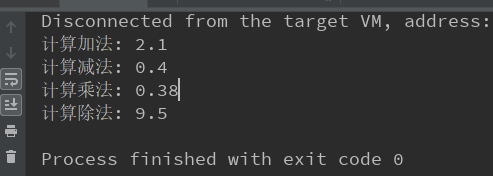注意1：BigDecimal的运算结果都是返回了一个新的BigDecimal对象，并不是在原有的对象上进行操作。
public static void main(String[] args) {
BigDecimal a = BigDecimal.valueOf(5);
System.out.println("a的地址：" + System.identityHashCode(a));
System.out.println("计算后a的地址：" + System.identityHashCode(a));
}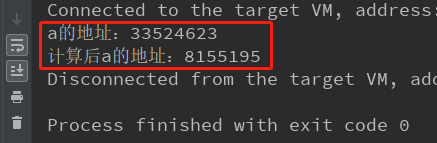注意2：使用divide除法函数除不尽，出现无线循环小数的时候，就需要使用另外精确的小数位数以及舍入模式，不然会出现报错。例如：
public static void main(String[] args) {
BigDecimal a = BigDecimal.valueOf(10), b = BigDecimal.valueOf(3);
System.out.println(a.divide(b));
}

// 该程序运行会出现以下错误
Exception in thread "main" java.lang.ArithmeticException: Non-terminating decimal expansion; no exact representable decimal result.
at java.math.BigDecimal.divide(BigDecimal.java:1690)
at com.fivesix._05_bigdecimal.Demo01.main(Demo01.java:31)

解决方法如下（此处舍入模式使用四舍五入的方式，其他模式在该文章后面有讲解）：
public static void main(String[] args) {
BigDecimal a = BigDecimal.valueOf(10), b = BigDecimal.valueOf(3);
System.out.println(a.divide(b, 3, BigDecimal.ROUND_HALF_UP));
}
// 该程序运行后输出：
3.33

4、BigDecimal比较大小
public int compareTo(BigDecimal val)

BigDecimal类提供的比较值的方法，注意比较的两个值均不能为空。 a.compareTo(b)得到结果 1， 0， -1。
比较结果描述1a 大于b0a 等于b-1a 小于b
代码示例：
public static void main(String[] args) {
BigDecimal a = BigDecimal.valueOf(1);
BigDecimal b = BigDecimal.valueOf(2);
BigDecimal c = BigDecimal.valueOf(1);
BigDecimal d = BigDecimal.ZERO;
System.out.println("1和2比较结果：" + a.compareTo(b));
System.out.println("1和1比较结果：" + a.compareTo(c));
System.out.println("1和0比较判断：" + (a.compareTo(d) > 0) );
}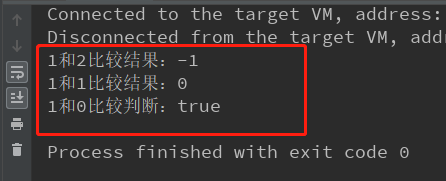5、BigDecimal保留两位小数及舍入模式
public BigDecimal setScale(int newScale, int roundingMode)

用于格式化小数的方法，第一个值表示保留几位小数，第二个值表示格式化的类型。 8种类型如下：
格式化类型描述ROUND_DOWN舍弃多余位数，如1.55会格式化为1.5，-1.55会格式化为-1.5ROUND_UP进位处理，如1.52会格式化为1.6，-1.52会格式化为-1.6ROUND_HALF_UP四舍五入，如果舍弃部分>= .5，则进位ROUND_HALF_DOWN五舍六入，如果舍弃部分> .5，则进位ROUND_CEILING正无穷大方向舍入模式。如果值为正数，则与ROUND_UP模式相同；如果值为负数，则与ROUND_DOWN模式相同ROUND_FLOOR负无穷大方向舍入模式。如果值为正数，则与ROUND_DOWN模式相同；如果值为负数，则与ROUND_UP模式相同ROUND_UNNECESSARY确认值的小数位数是否与传入第一个参数（保留小数的位数）相等，如果符合则返回值，如果不符抛出异常ROUND_HALF_EVEN如果舍弃部门左边的数字为奇数，则与ROUND_HALF_UP模式相同，如果为偶数则与ROUND_HALF_DOWN模式相同
代码示例：
public static void main(String[] args) {
BigDecimal a = BigDecimal.valueOf(5.445);
System.out.println("5.445舍弃多余位数：" + a.setScale(2, BigDecimal.ROUND_DOWN));
System.out.println("5.445进位处理：" + a.setScale(2, BigDecimal.ROUND_UP));
System.out.println("5.445四舍五入(舍弃部分>= .5,进位)：" + a.setScale(2, BigDecimal.ROUND_HALF_UP));
System.out.println("5.445四舍五入(舍弃部分未> .5,舍弃)：" + a.setScale(2, BigDecimal.ROUND_HALF_DOWN));
System.out.println("5.446四舍五入(舍弃部分> .5,进位)：" + BigDecimal.valueOf(5.446).setScale(2, BigDecimal.ROUND_HALF_DOWN));
}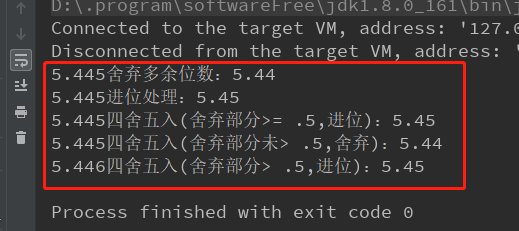6、BigDecimal其他方法及常量
代码类型描述BigDecimal.ZERO常量初始化一个为0的BigDecimal对象BigDecimal.ONE常量初始化一个为1的BigDecimal对象BigDecimal.TEN常量初始化一个为10的BigDecimal对象public BigDecimal abs()方法求绝对值，不管正数还是负数，都得到正数public BigDecimal negate()方法求相反数，正变负，负变正public BigDecimal pow(int n)方法求乘方，如BigDecimal.valueOf(2).pow(3)的值为8public BigDecimal max(BigDecimal val)方法两值比较，返回最大值public BigDecimal min(BigDecimal val)方法两值比较，返回最小值
展开全文java 后端
• php中文网最新课程每日17点准时技术干货分享这篇文章主要介绍了PHP保留两位小数的几种方法，在一些商品价格上也经常遇到这样的需求，今天小编给大家分享的实例代码,需要的朋友可以参考下代码如下所示：\$num = 10....
• java保留两位小数问题： 一： 四舍五入(四舍五入形式保留两位小数，注意模式ROUND_HALF_UP) double f = 2345.2345; BigDecimal b = new BigDecimal(f); double f1 = b.setScale(2, BigDecimal.ROUND_HALF_UP).double...
• * 保留两位小数 */ @Test public void test2(){ BigDecimal bd=null; // 始终显示两位小数 double[] num = {3.4567, 3, 0.4567, 2343.333, 133, 3.1415}; for (double n : num) { bd = new BigDecimal(n); ...java
• BigDecimal 保留两位小数[/b] 今天在项目中遇到这样的一个需求，计算的结果保留两位小数点，不要求进行四舍五入。 直接上代码了，没有什么好说的。 [code="java"] BigDecimal decimal = new ...java date timestamp
• // 四舍五入 BigDecimal value = new BigDecimal(object.toString()).setScale(2,BigDecimal.ROUND_HALF_UP);... // 不足两位小数补0 DecimalFormat decimalFormat = new DecimalFormat("0.00#"); ...
• 作者：RaphetS第一种方法使用DecimalFormat类举个例子，假如我们需要保留两位小数，我们可以这样写DecimalFormat df = new DecimalFormat("0.00");测试如下：double d = 0.200;DecimalFormat df = new DecimalFormat...
• **问题：**将两个long类型的数相除后转换为BigDecimal类型并保留两位小数。 思路： 1.先将long转换为double类型再相除 2.相除之后再转换为BigDecimal类型 3.最后是设置小数位数，并设置两位小数后面的数的处置方式。...java
• //保留两位小数并展示千分位符 DecimalFormat df1 = new DecimalFormat("##,##0.00"); System.out.println(df1.format(0.2));// 0.20 System.out.println(df1.format(0.235));// 0.24 System.out.println(df1....java
• java保留两位小数问题： 方式一(四舍五入形式保留两位小数，注......